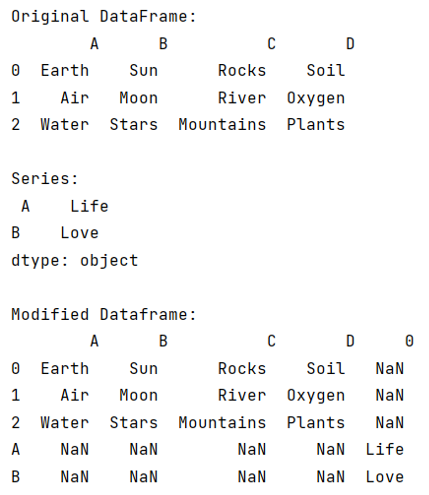# How to Merge a Series and DataFrame?

Given a Series and DataFrame, we have to merge them. By Pranit Sharma Last updated : September 24, 2023

Both DataFrame and series are the two main data structures of the pandas library. A Series in pandas contains a single list that can store heterogeneous types of data, because of this, a series is also considered as a 1-dimensional data structure.

On the other hand, DataFrame is a 2-dimensional data structure that contains multiple lists of the heterogeneous type of data. DataFrame can contain multiple series or it can be considered as a collection of series.

When we analyze a series, each value can be considered as a separate row of a single column. In contrast, when we analyze a DataFrame, we have multiple columns and multiple rows.

Both series and DataFrame can be created either with the list or with the help of a dictionary, in the case of series, there will be only one key in the dictionary but there may be multiple keys in the case of DataFrame.

## Merging a Series and DataFrame

To merge Series and DataFrame, we will first convert Series into DataFrame by using series.to_frame() method and then we will merge the two frames by using pandas.concat() method.

Note

To work with pandas, we need to import pandas package first, below is the syntax:

```import pandas as pd
```

Let us understand with the help of an example,

## Python program to merge a series and dataframe

```# Importing pandas package
import pandas as pd

# Creating a dictionary
d = {
'A':['Earth','Air','Water'],
'B':['Sun','Moon','Stars'],
'C':['Rocks','River','Mountains'],
'D':['Soil','Oxygen','Plants']
}

# Creating a DataFrame
df = pd.DataFrame(d)

# Creating a Series
s = pd.Series({'A':'Life','B':'Love'})

# Display original DataFrame
print("Original DataFrame:\n",df,"\n")

# Display Series
print("Series:\n",s,"\n")

# Converting series into DataFrame
s_f = s.to_frame()

# Encapsulating both frames in a list
frames = [df,s_f]

# concatenating series and dataframe
df = pd.concat(frames)

# Display result
print("Modified Dataframe:\n",df)
```

### Output

The output of the above program is: